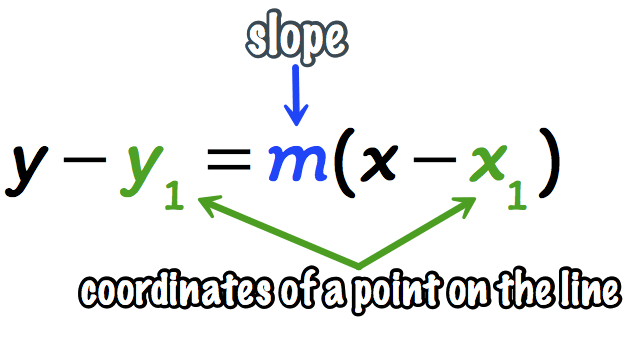# How do you find the slope of y=3+2 (x-1)?

Aug 2, 2018

The slope is 2.

#### Explanation:

This equation is in point-slope form, shown here:We know that the slope is the value multiplied by $\left(x - {x}_{1}\right)$, so in our equation the slope is 2 .

Hope this helps!

Aug 2, 2018

$2$

#### Explanation:

Recall point-slope form

$y - {y}_{1} = m \left(x - {x}_{1}\right)$, where ${x}_{1}$ and ${y}_{1}$ are points, and $m$ is our slope.

We can subtract $3$ from both sides to now get

$y - 3 = 2 \left(x - 1\right)$

We see that our coefficient on the $x$ term is $2$. This is our slope.

Hope this helps!